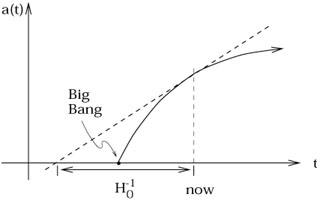# Shouldn't the estimate of the universe's age be higher, not lower, after the attractive strength of gravity is taken into account?

From 'Fundamentals: Ten Keys to Reality', by Frank Wilczek :

"Running the movie of cosmic history backward in our minds, we found the galaxies all coming together to meet at a definite time. When did it happen? To calculate how long ago, we simply divide the distance a galaxy must travel by the speed at which it's moving. (Since a galaxy's speed is proportional to its distance according to Hubble's observations, we'll find the same result consistently whichever galaxy we choose.) Doing that, we estimate that galaxies were all smushed together about 20 billion years ago. More accurate calculations, which include how the velocities change over time due to gravity, yield a somewhat smaller result. Today's best estimate is that 13.8 billion years have elapsed since the big bang."

Since gravity is an attractive force, partially offsetting the expansion of the universe, shouldn't the estimated age be higher than the initial 20 billion years, not lower?

For instance, if astronomers, somehow, discovered that there was even more matter in the universe than estimated, wouldn't that cause them to revise the estimated age of the universe upwards, not downwards?

In other words, with gravity partially resisting and slowing down the expansion, wouldn't it have taken that much longer for the universe to reach its current size?The averaged distances and speeds of nearby galaxies give us the slope of the curve at the horizontal position marked "now". If you assume they've always moved at that speed, you get the scale factor indicated by the dotted line, while if you take into account that they're slowing down, you get the solid curve, which reaches $$0$$ (intersects the $$t$$ axis) at a more recent time.
Wilczek is wrong when he says that linear extrapolation gives an age of 20 billion years. It actually gives about 14 billion years, close to the correct value. This is more or less an accident: it's only true during the transition from matter-dominated decelerating expansion to dark-energy-dominated accelerating expansion, which happens to be happening now. In old matter-dominated cosmological models (predating the discovery of dark energy), linear extrapolation gives you about $$1.5$$ times the correct age, so it's possible that Wilczek got 20 billion by multiplying a modern estimate of the age by a ratio from an obsolete model.